# NCERT Exemplar Class 11 Maths Solutions for Chapter 15 - Statistics

NCERT Exemplar Class 11 Maths Chapter 15 Statistics are provided here for 11th standard students to make them prepare for the exam and score good marks. Practice from class 11 Maths chapter 15 statistics exemplar problems and solutions prepared by our subject experts in accordance with CBSE syllabus (2018-2019) to score well in exams.

Students can download the pdf format of NCERT exemplars and practice it offline. They can use it as a reference tool while solving difficult maths problems.

## Class 11 Maths NCERT Exemplar Problems for Statistics

One of the major branches of mathematics which deal with the collection of data along with the organization, analysis, interpretation and presentation is termed as Statistics. In mathematics, statisticians can collect data from different experimental designs and survey samples. Different kind of studies like an observational study and experimental study are used for taking measurements and manipulating the values of the measurement.

In chapter 15 exemplars, students will get solved questions based on Statistics and sub-topics given below;

• Statistics, Mean and median of the data
• Measures of Dispersion, based on Range, Quartile deviation,
• Mean deviation, Standard deviation Mean deviation for grouped and ungrouped data
• Discrete frequency distribution and Continuous frequency distribution and their standard deviation Also, learn the shortcut method for calculating mean deviation about mean
• Shortcut method to find variance and standard deviation
• Comparison of two frequency distributions with the same mean

Students of are provided with pdf of 11th class Maths NCERT solutions, notes and question papers for Maths subject, as a learning source, to prepare for exams. Also, it is advised for them to solve sample papers and previous year question papers to understand the marking scheme and question pattern for chapter Statistics.

Get the NCERT Exemplar for Class 11 Maths Chapter 15 Statistics PDF as a download below: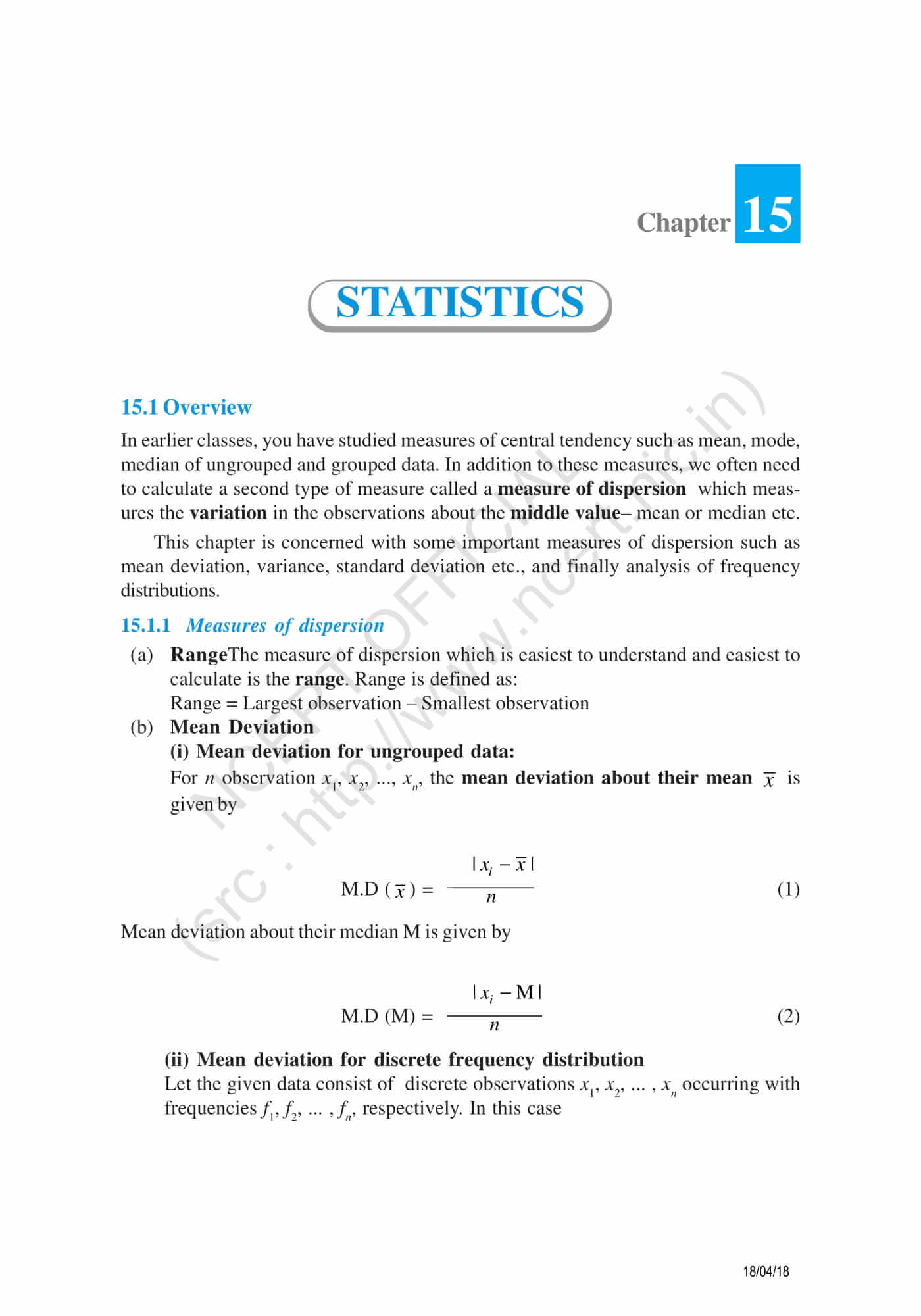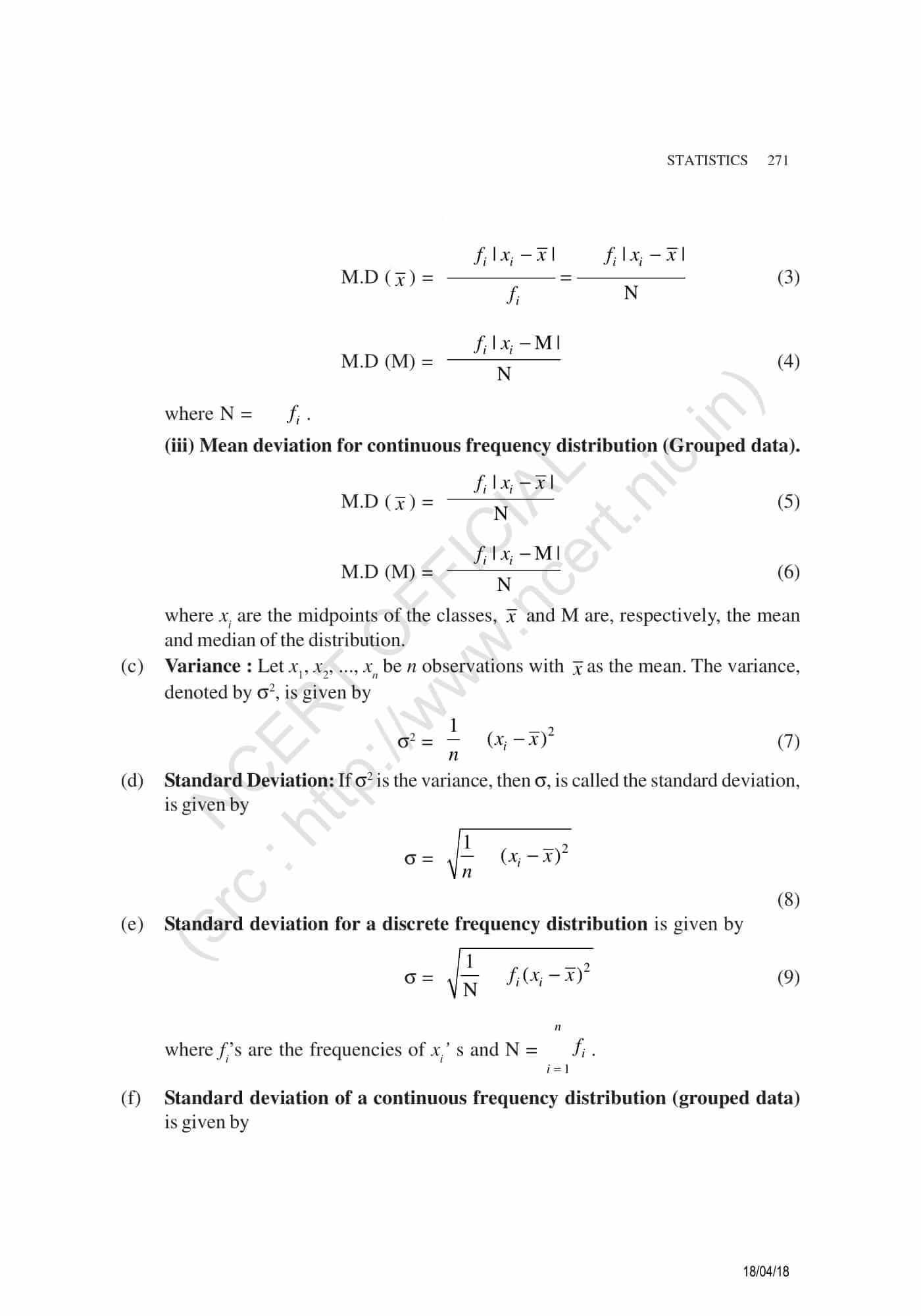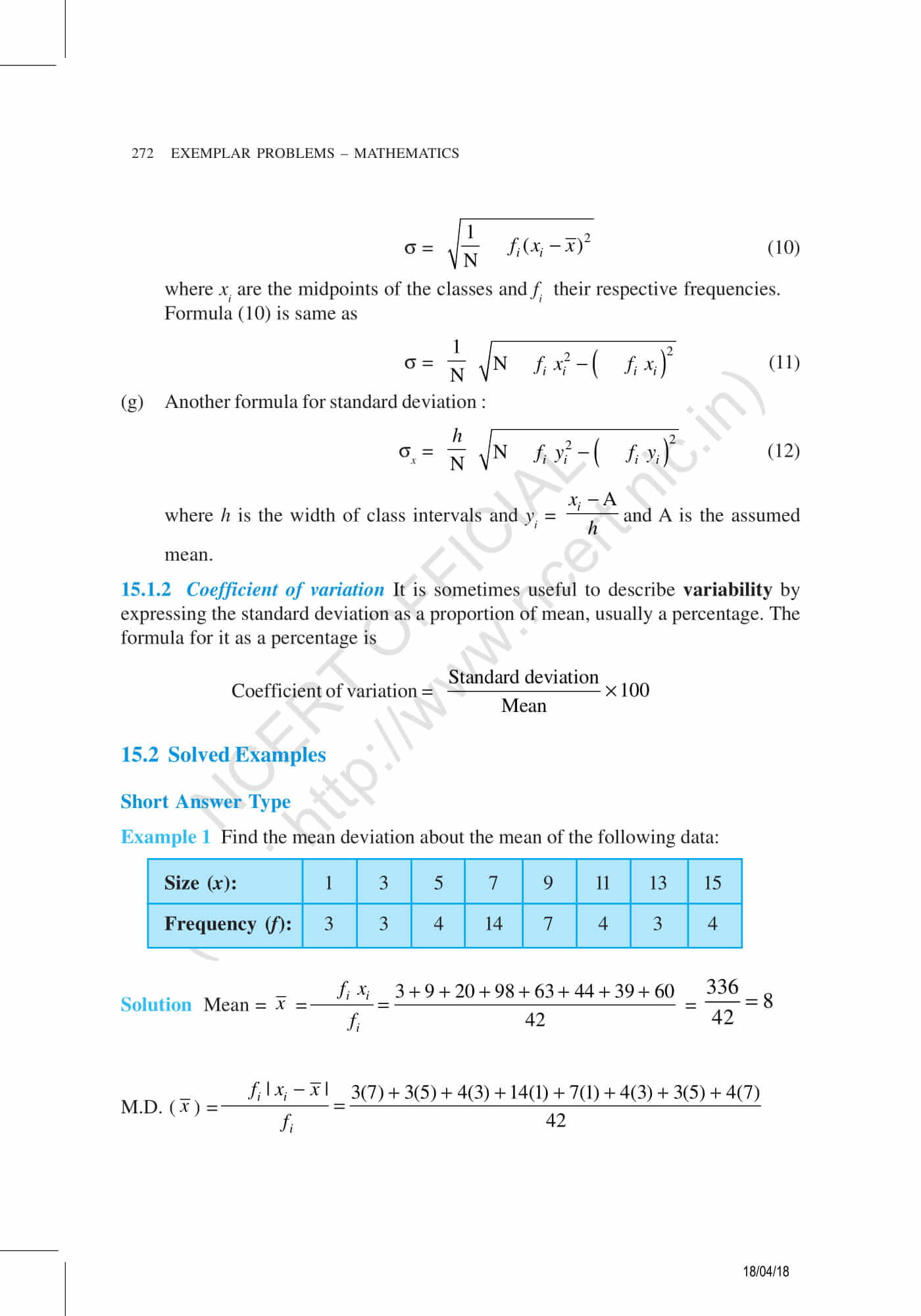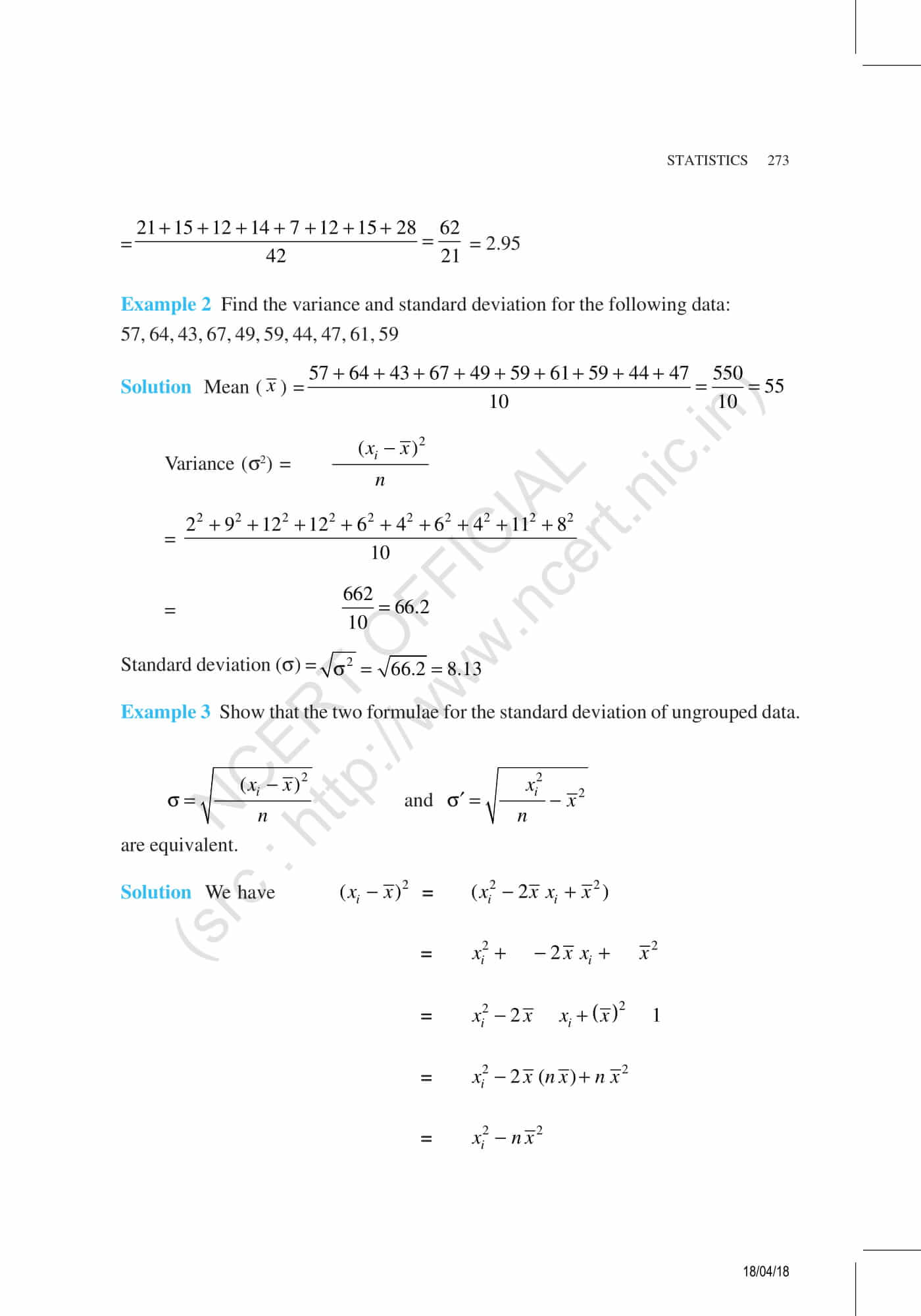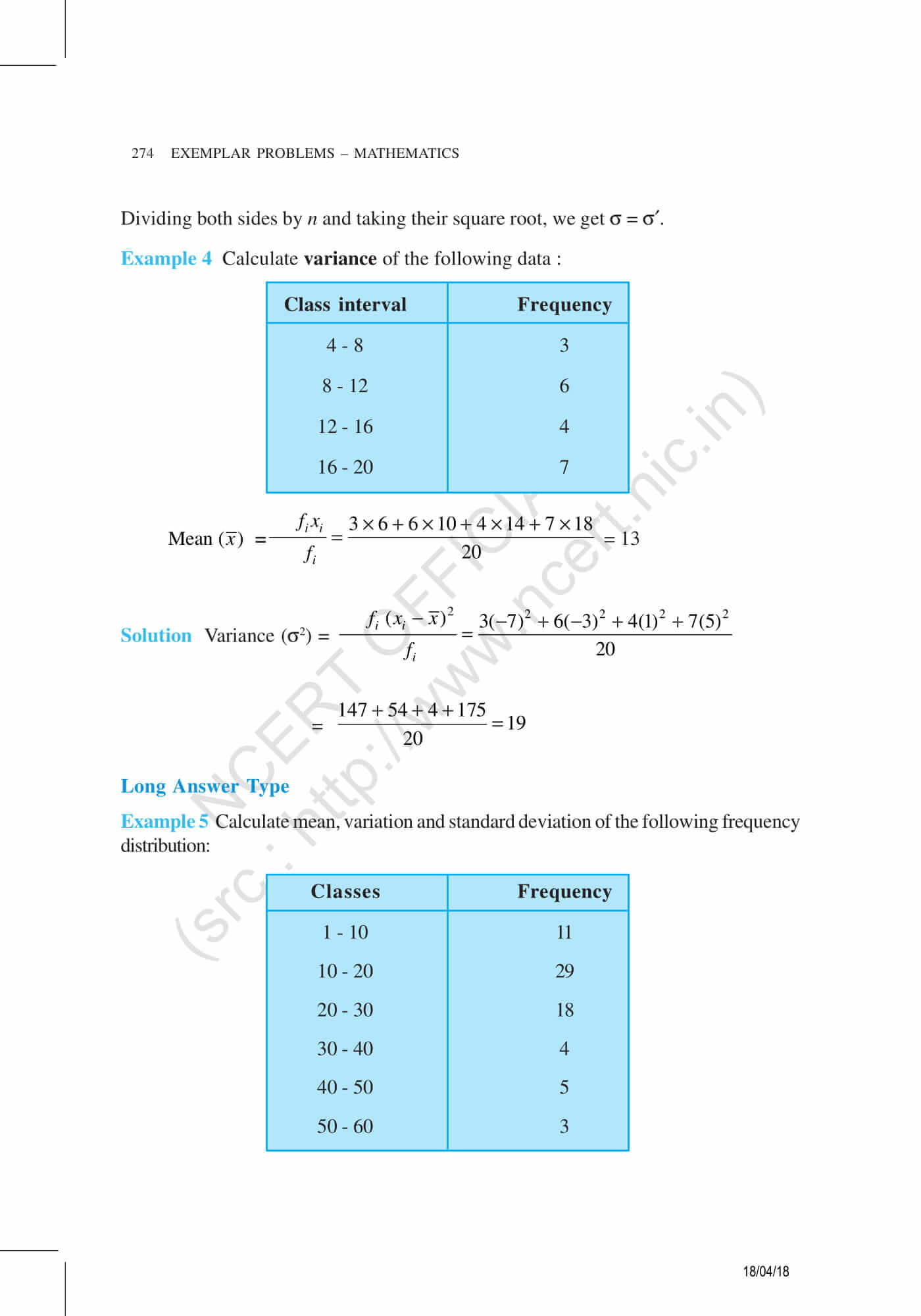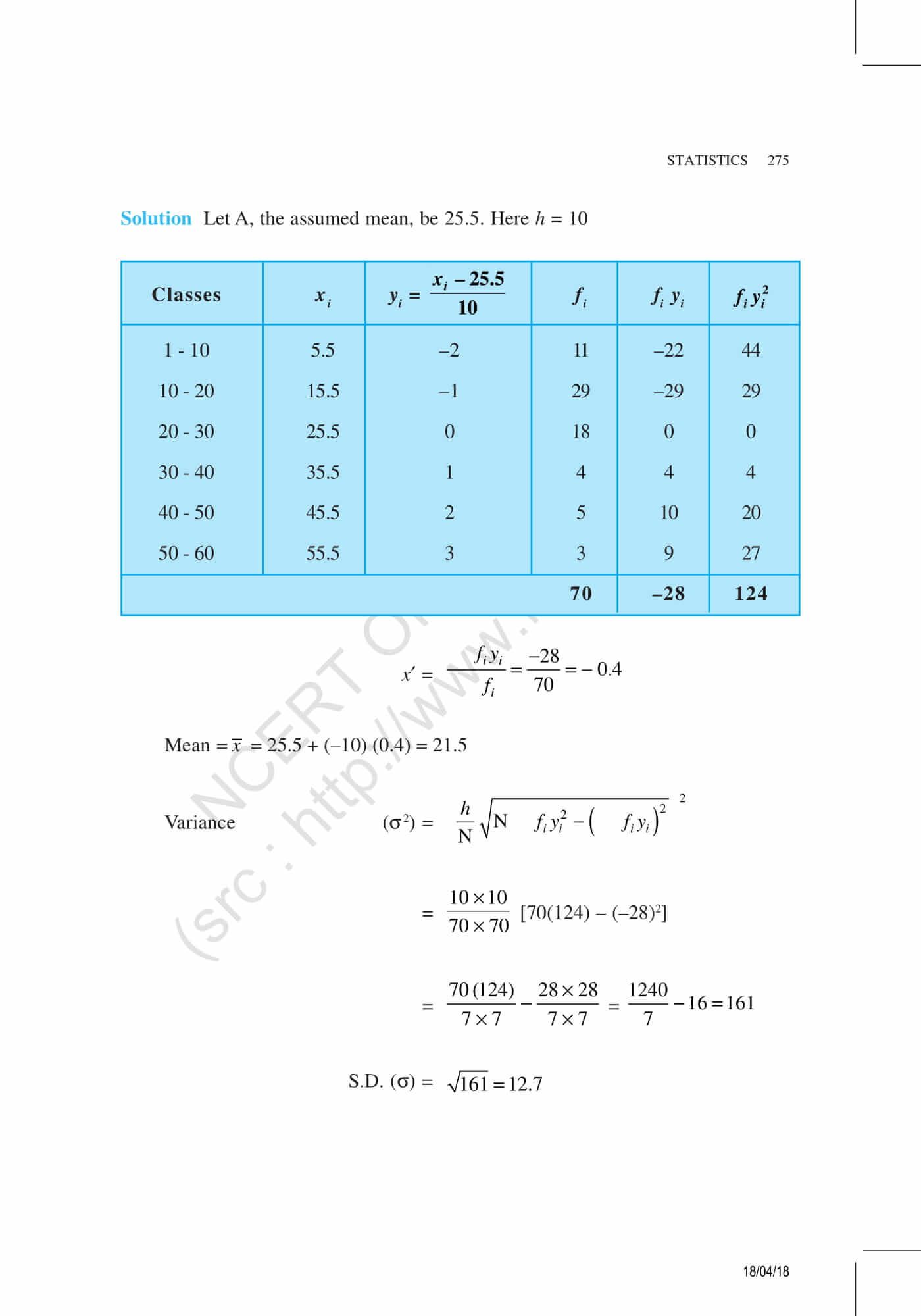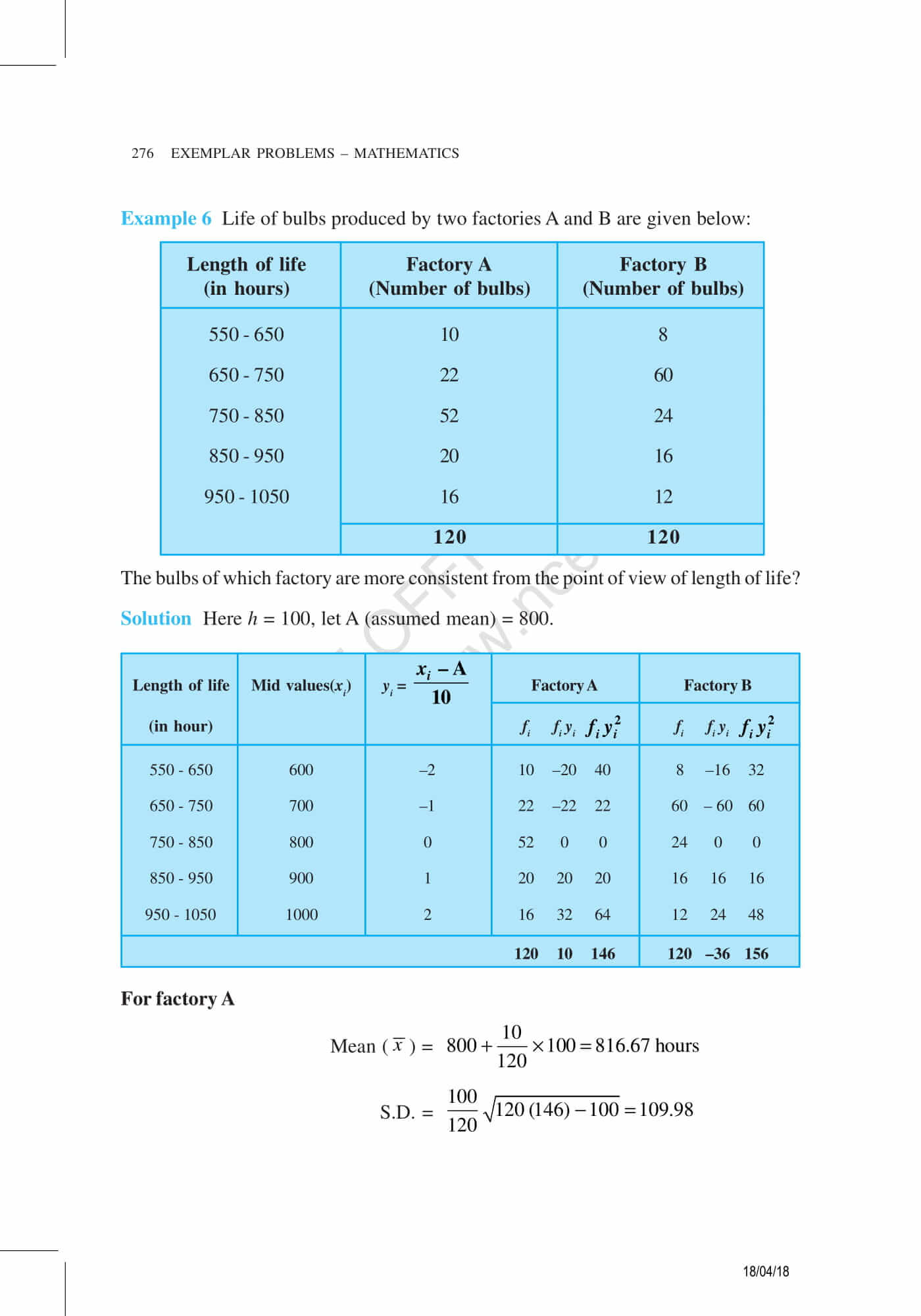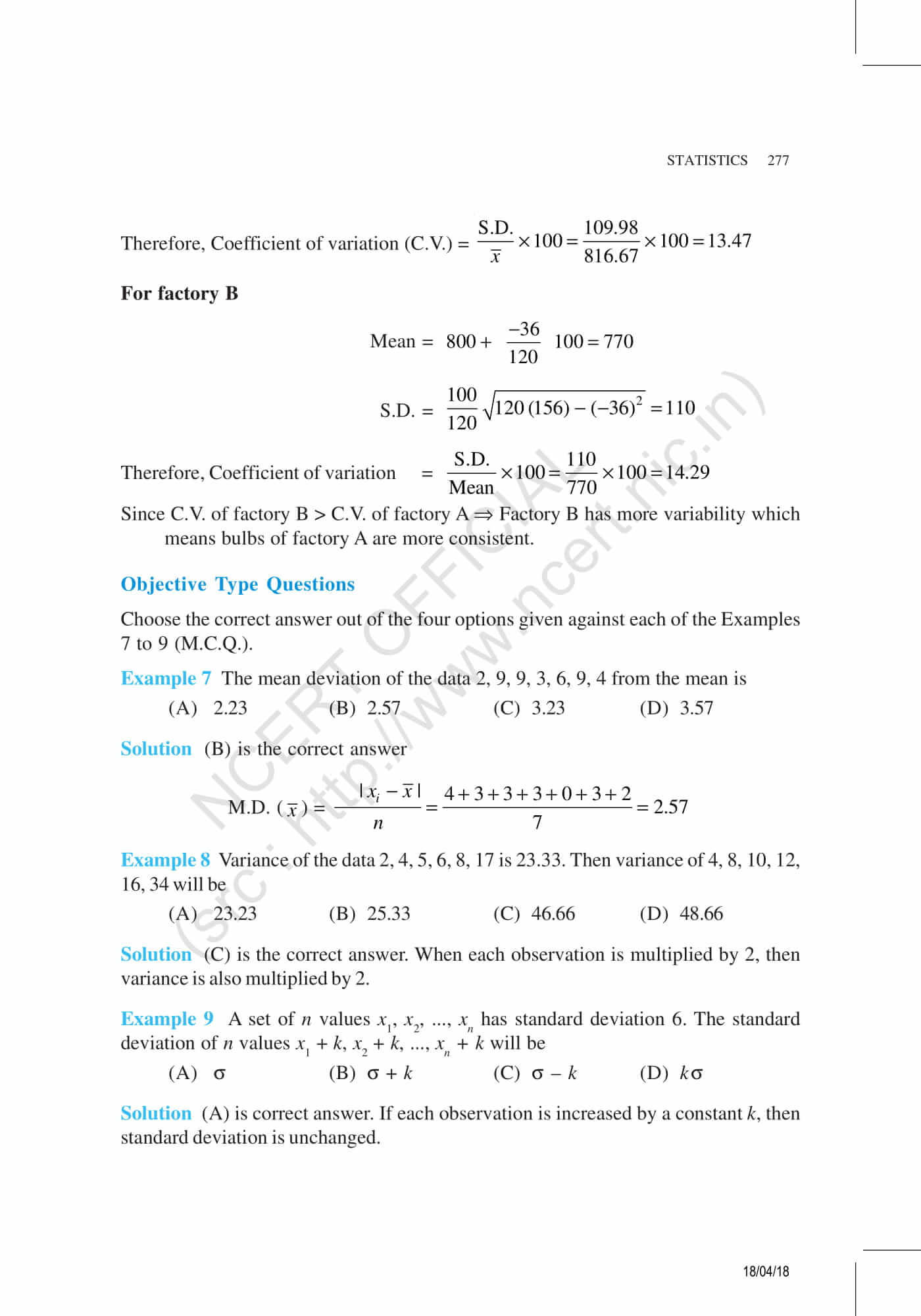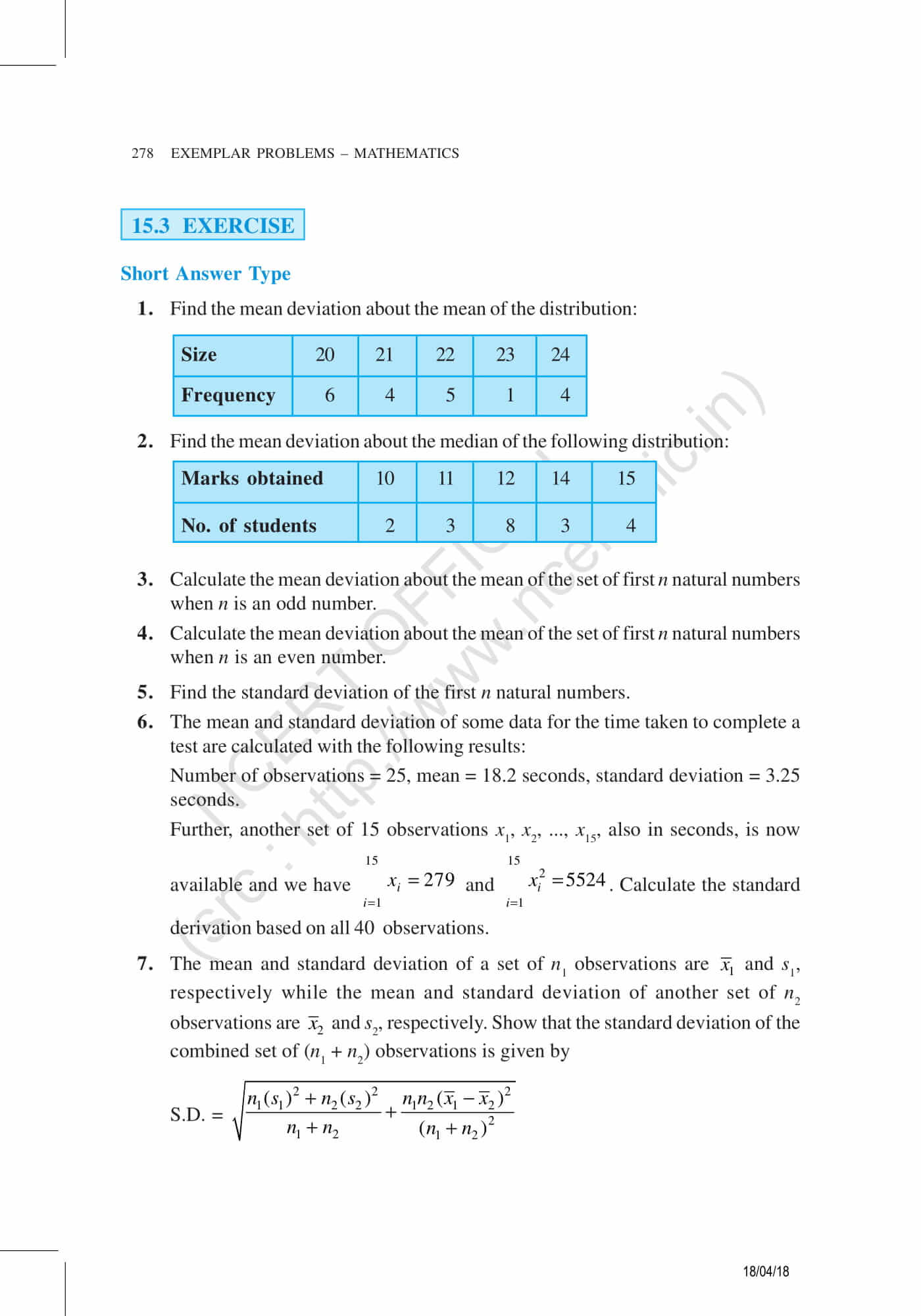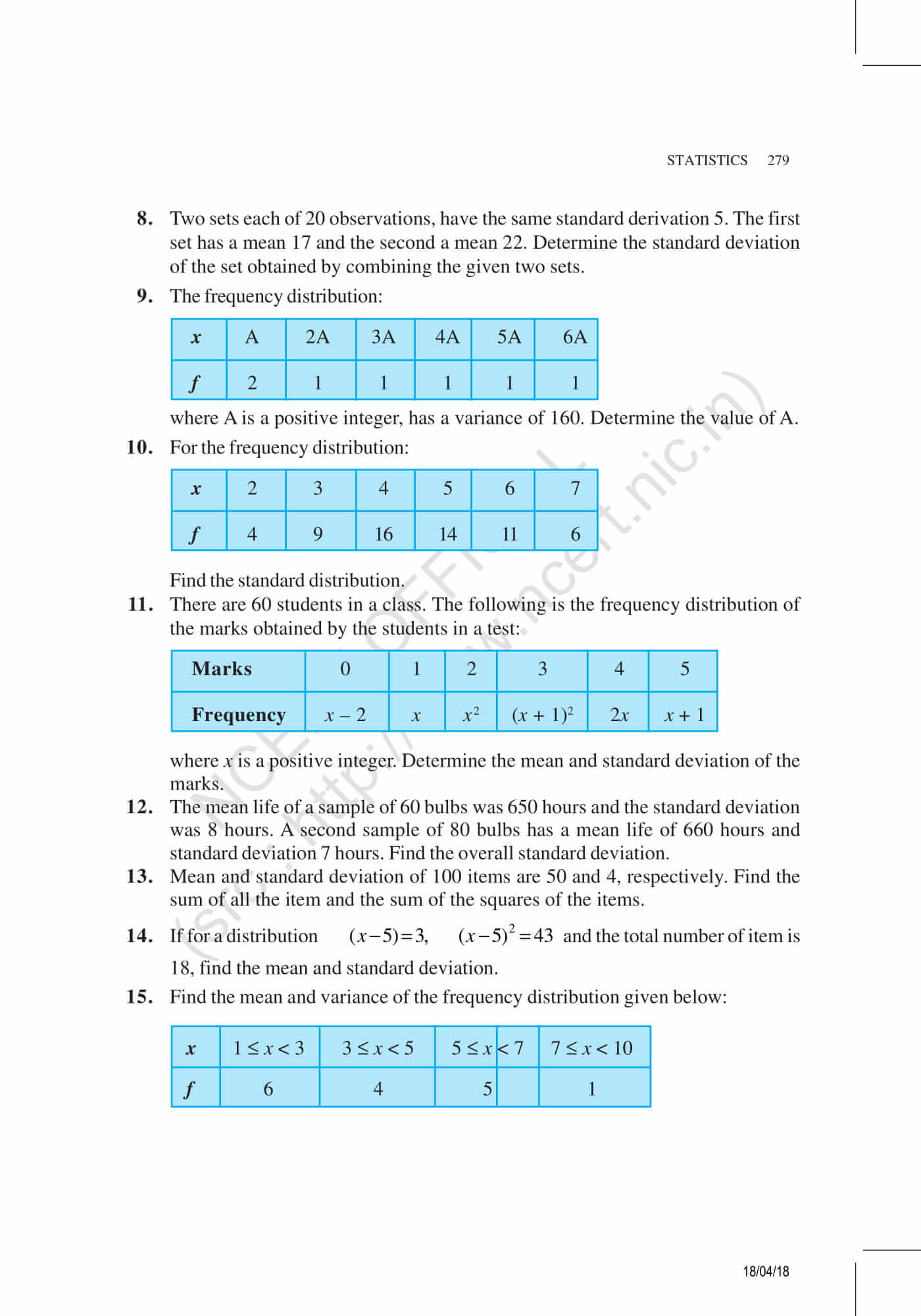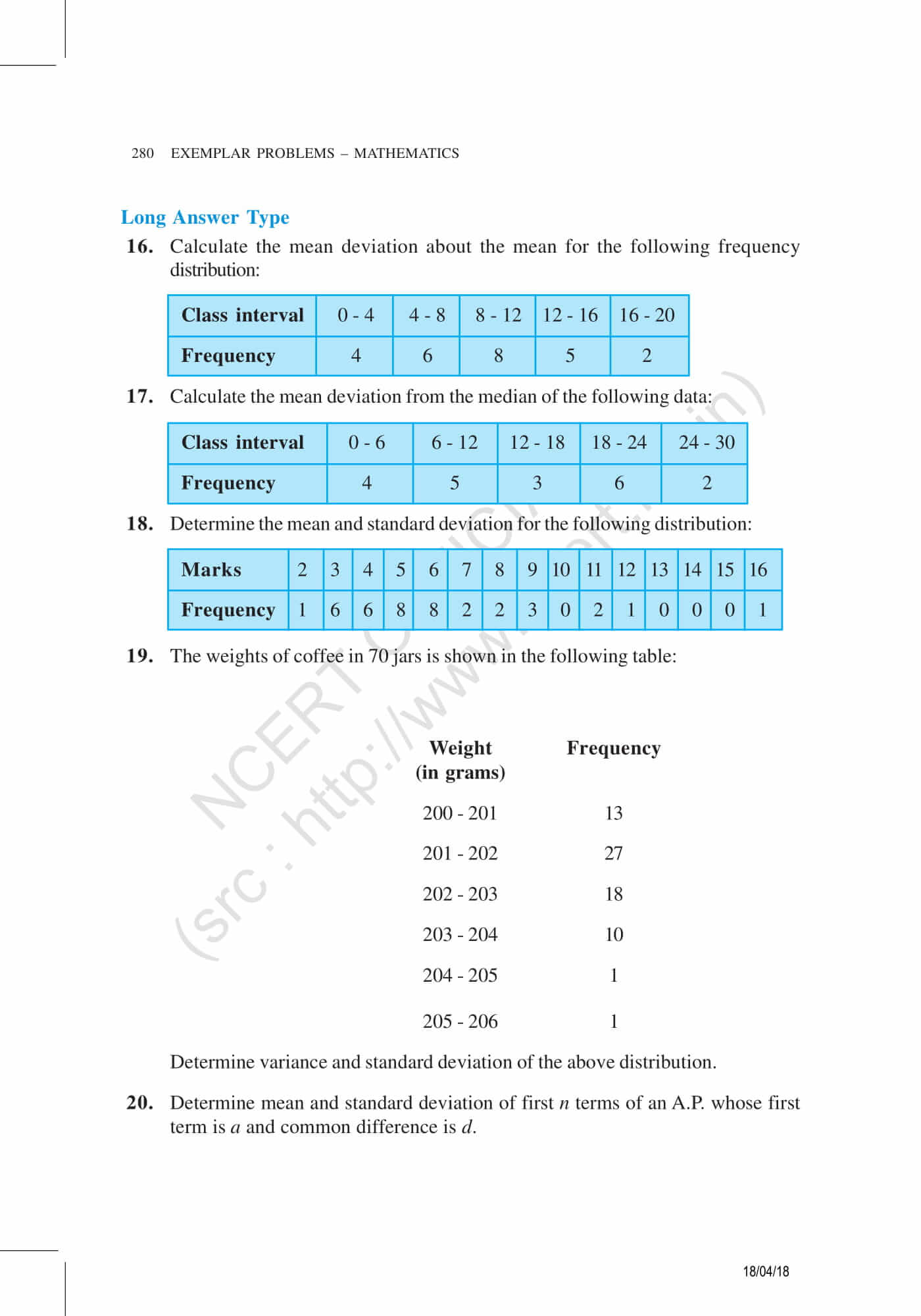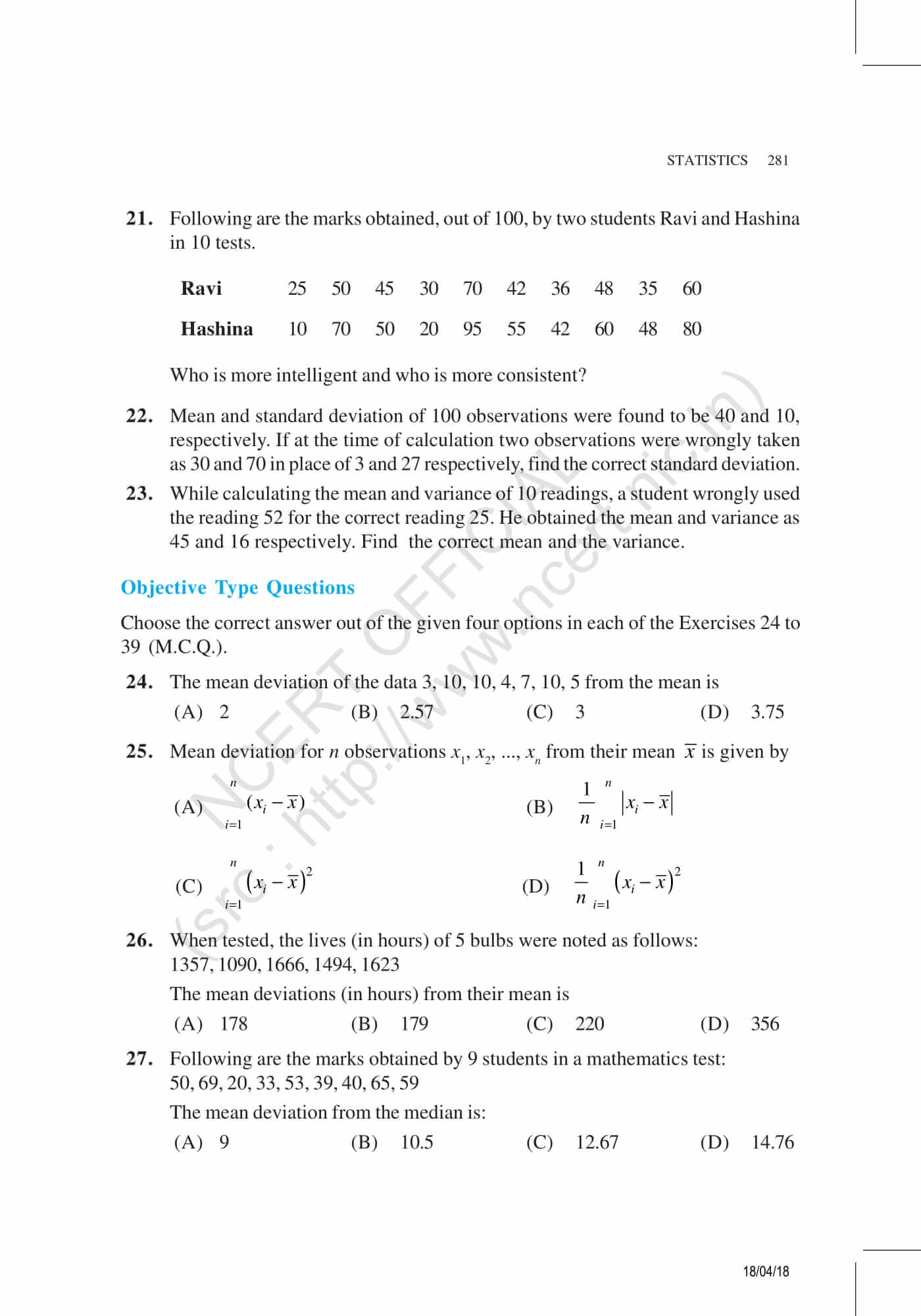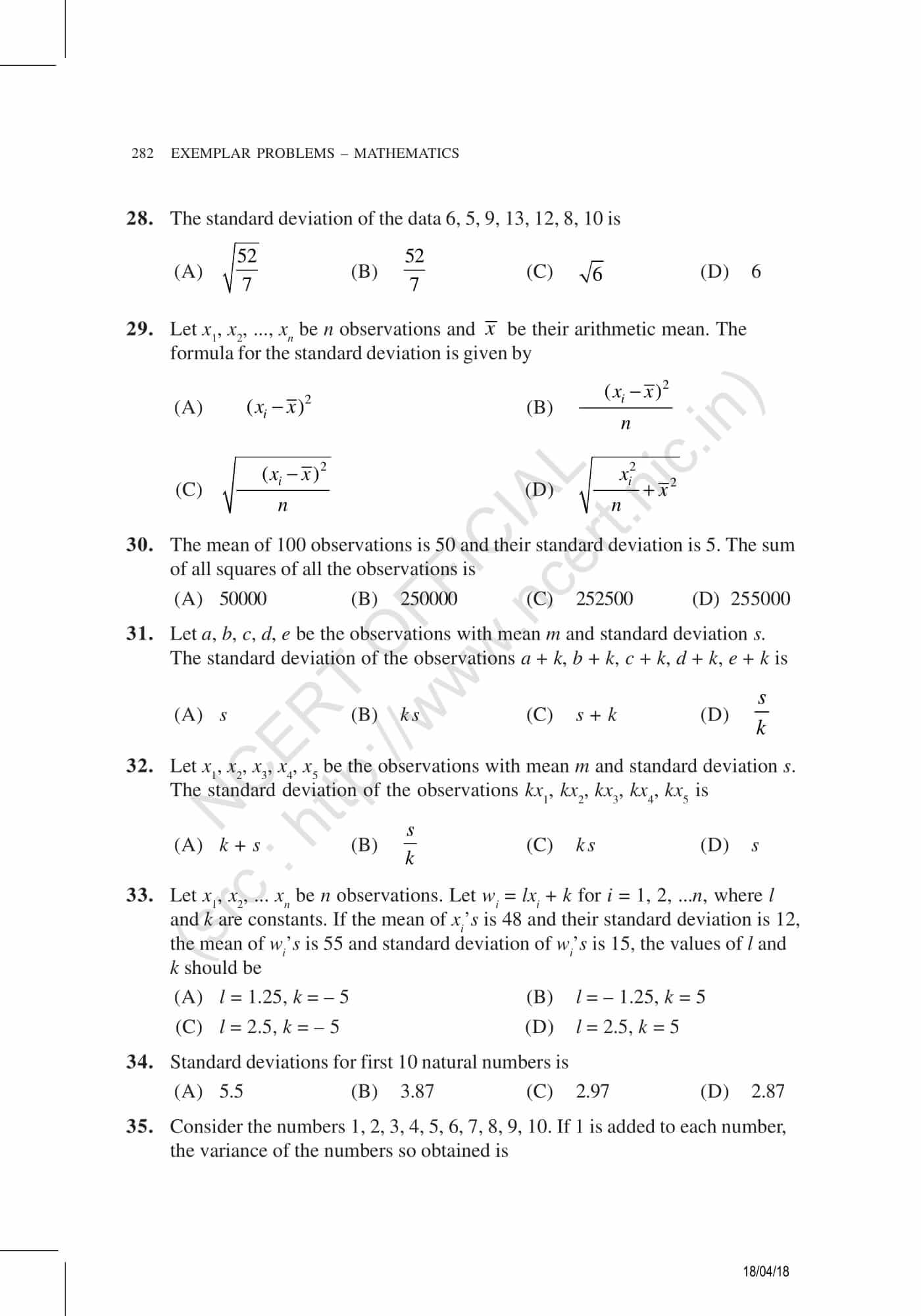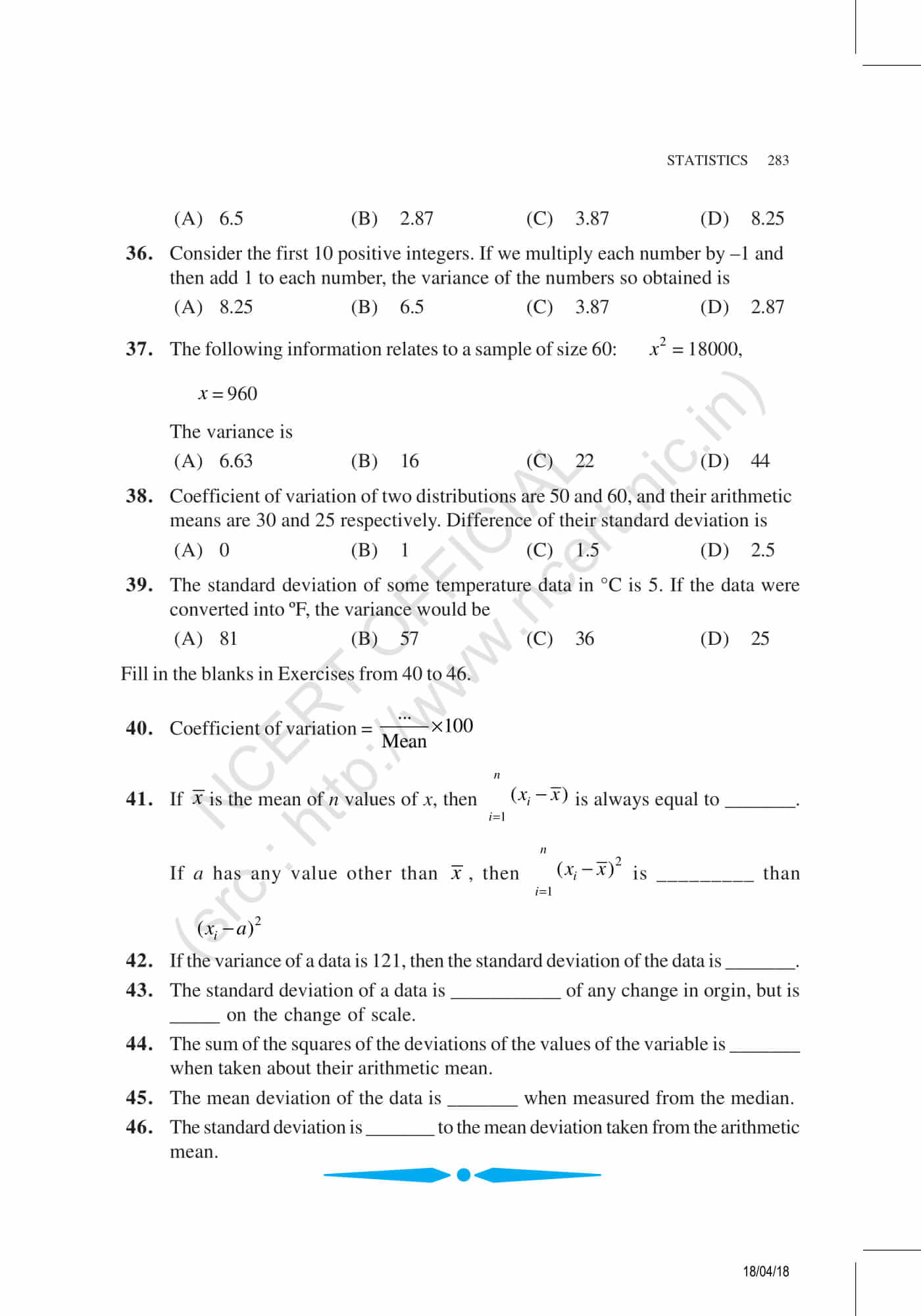BYJU’S provides new learning methods and preparation tips to help students to solve the maths problems easily and quickly. Download the pdf of all the chapters to solve Maths exemplar problems for class 11.

Also, we have educational videos to help students visualize different types of concepts such as statistics, which can be accessed by downloading BYJU’S- The learning app on your mobile or tablets.